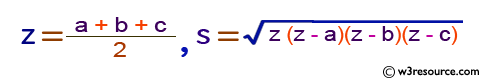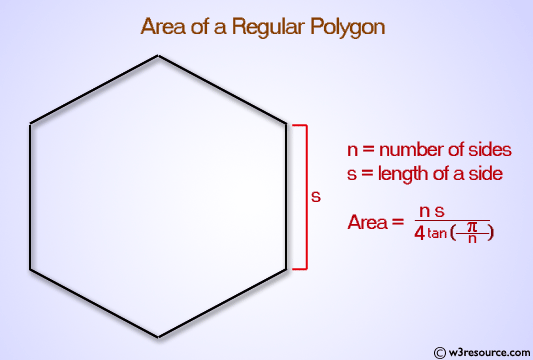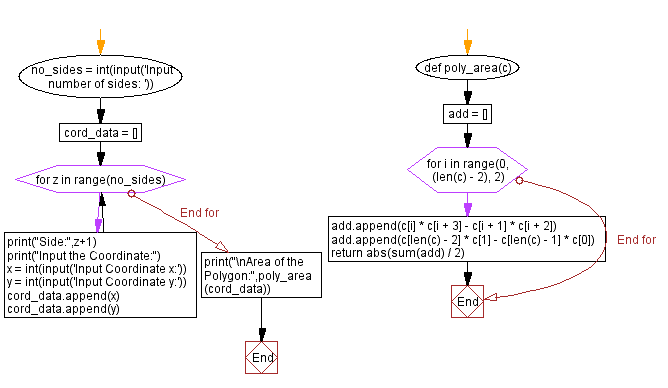﻿ Python: Compute the area of the polygon - w3resource# Python: Compute the area of the polygon

## Python Basic - 1: Exercise-59 with Solution

A convex polygon is a simple polygon in which no line segment between two points on the boundary ever goes outside the polygon. Equivalently, it is a simple polygon whose interior is a convex set. In a convex polygon, all interior angles are less than or equal to 180 degrees, while in a strictly convex polygon all interior angles are strictly less than 180 degrees.

Write a Python program that compute the area of the polygon . The vertices have the names vertex 1, vertex 2, vertex 3, ... vertex n according to the order of edge connections.

Input:
Input number of sides: 5
Side: 1
Input the Coordinate:
Input Coordinate x: 1
Input Coordinate y: 0
Side: 2
Input the Coordinate:
Input Coordinate x: 0
Input Coordinate y: 0
Side: 3
Input the Coordinate:
Input Coordinate x: 1
Input Coordinate y: 1
Side: 4
Input the Coordinate:
Input Coordinate x: 2
Input Coordinate y: 0
Side: 5
Input the Coordinate:
Input Coordinate x: -1
Input Coordinate y: 1
Area of the Polygon: 0.5Pictorial Presentation:Sample Solution:

Python Code:

``````def poly_area(c):
for i in range(0, (len(c) - 2), 2):
add.append(c[i] * c[i + 3] - c[i + 1] * c[i + 2])
add.append(c[len(c) - 2] * c - c[len(c) - 1] * c)
no_sides = int(input('Input number of sides: '))
cord_data = []
for z in range(no_sides):
print("Side:",z+1)
print("Input the Coordinate:")
x = int(input('Input Coordinate x:'))
y = int(input('Input Coordinate y:'))
cord_data.append(x)
cord_data.append(y)
print("\nArea of the Polygon:",poly_area(cord_data))
``````

Sample Output:

```Input number of sides:  5
Side: 1
Input the Coordinate:
Input Coordinate x: 1
Input Coordinate y: 0
Side: 2
Input the Coordinate:
Input Coordinate x: 0
Input Coordinate y: 0
Side: 3
Input the Coordinate:
Input Coordinate x: 1
Input Coordinate y: 1
Side: 4
Input the Coordinate:
Input Coordinate x: 2
Input Coordinate y: 0
Side: 5
Input the Coordinate:
Input Coordinate x: -1
Input Coordinate y: 1

Area of the Polygon: 0.5
```

Flowchart:## Visualize Python code execution:

The following tool visualize what the computer is doing step-by-step as it executes the said program:

Python Code Editor:

Have another way to solve this solution? Contribute your code (and comments) through Disqus.

What is the difficulty level of this exercise?

Test your Programming skills with w3resource's quiz.

﻿

## Python: Tips of the Day

Iterating over dictionaries using 'for' loops:

I am a bit puzzled by the following code: d = {'x': 1, 'y': 2, 'z': 3} for key in d: print key, 'corresponds to', d[key] What I don't understand is the key portion. How does Python recognize ...

key is just a variable name.

```for key in d:
```

For Python 3.x:

```for key, value in d.items():
```

For Python 2.x:

```for key, value in d.iteritems():
```

To test for yourself, change the word key to poop.

In Python 3.x, iteritems() was replaced with simply items(), which returns a set-like view backed by the dict, like iteritems() but even better. This is also available in 2.7 as viewitems().

The operation items() will work for both 2 and 3, but in 2 it will return a list of the dictionary's (key, value) pairs, which will not reflect changes to the dict that happen after the items() call. If you want the 2.x behavior in 3.x, you can call list(d.items()).

Ref: https://bit.ly/37dm0Qo